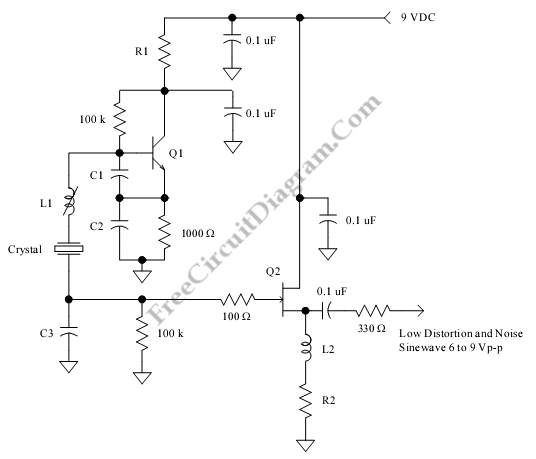# Low Distortion Crystal OscillatorThis is a Low Distortion Crystal Oscillator circuit. This circuit generate a sinewave that has low phase noise and distortion. This circuit can be used to perform a crystal with less than 1mV dissipated in crystal. The crystal is used to filter the signal current. If the impedance loads is low, the JFET will drive the impedance. when the loads is about 50ohm, it will better if an emitter follower combined with a voltage step-down transformer or matching network for further buffering. Here is the circuit:The value of C3 determined the output voltage, if the lower output voltage is required, the C3’s value should be increased and decrease the value of C3 when the larger output voltage is needed. If overtone crystal is used, a choke should replace the 1K emitter resistor. This choke must be resonate with C2 at a frequency slightly above the fundamental frequency for third  overtone  crystals. When uses the high-Q overtone crystal, the value of C3 should be lower because the High-Q  overtone  crystals should be driven at much lower levels than  fundamental  crystals. Beside that, the output level should be set as low as possible.

If the crystal’s  rated  power or current is  known, the drive level can be measured. To measure drive level temporarily connect a 100 ohm across C3 and measure the signal level on the source of the FET. The crystal current is determined by V/100. [Circuit’s schematic diagram source: techlib.com]